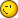# Derivation Of Composite Function

8 replies to this topic

### #1Abbe

Abbe

Newbie

•• Members
•• 4 posts

• Calculators:

Posted 16 January 2006 - 10:00 AM

I tried derivation of sin^3(2*x) like diff(sin^3(2*x)) and CP 300 return error "Incorect Argument". Can anybody help me with that syntax please<

### #2Guest_Guest_*

Guest_Guest_*
•• Guests

Posted 16 January 2006 - 02:58 PM

I tried derivation of sin^3(2*x) like diff(sin^3(2*x)) and CP 300 return error "Incorect Argument". Can anybody help me with that syntax please<

Your term sin^3(2x) is wrong. It has to be sin()...
Other than that, the syntax for the diff command is (Classpad Manual):

Syntax: diff(Exp/List)[,variable] [ ) ]
diff(Exp/List,variable,order[,a] [ ) ]
"a" is the point for which you want to determine the derivative.
"order" = 1 when you use the following syntax: diff(Exp/List[,variable][ ) ]. The default
variable is "x" when "variable" is omitted.
Example: To differntiate x^6 with respect to x:
diff(x^6)
6x^5
Example: To find the second derivative of x^6 with respect to x:
diff(x^6,x,2)
30x^4
Example: To find the second derivative of x^6 with respect to x at x=3
diff(x^6,x,2,3)
2430

### #3Abbe

Abbe

Newbie

•• Members
•• 4 posts

• Calculators:

Posted 16 January 2006 - 04:20 PM

But , can I derivate sin^3(2x)? There is no way to derive function of a function? Or which way I must wrote right syntaxe?

### #4Guest_Guest_*

Guest_Guest_*
•• Guests

Posted 16 January 2006 - 04:57 PM

But , can I derivate sin^3(2x)? There is no way to derive function of a function? Or which way I must wrote right syntaxe?

sin^3(2*x) is the problem. sin^3 isn't correct syntax.

### #5Gaumerie

Gaumerie

Newbie

•• Members
•• 28 posts

Posted 16 January 2006 - 05:04 PM

sin(2*x)^3 !!!!!!!!!!!!!!!!!!!!!!!!!!!!!

### #6Abbe

Abbe

Newbie

•• Members
•• 4 posts

• Calculators:

Posted 18 January 2006 - 07:51 AM

sin^3(2*3) IS NOT EQUAL sin(2*x)^3 !!!!!!!!!!!!!!!!!!!!!!!!!!!!! because in first case i square function sin^3 in second you square (2*x)^3
That is mathematical diferent function.

### #7Overlord

Overlord

Casio Technician

•• Moderator
•• 355 posts
• Gender:Male
• Location:Brussels - Belgium
• Interests:Math Researcher

• Calculators:
My head - C300 OS 3.00 - G100 Rom 1.02 - G65 - G60 - G25

Posted 18 January 2006 - 12:33 PM

What do you mean by sin^3 (2x) ?
sin(sin(sin(2x))) or (sin(2x))^3 ?

Note : What Gaumerie wrote is (sin(2x))^3 and not sin((2x)^3)

### #8Abbe

Abbe

Newbie

•• Members
•• 4 posts

• Calculators:

Posted 18 January 2006 - 12:59 PM

What do you mean by sin^3 (2x) ?
sin(sin(sin(2x))) or (sin(2x))^3 ?

Note : What Gaumerie wrote is (sin(2x))^3 and not sin((2x)^3)

It seems to me that (sin(2x))^3 is the right function.

### #9Kilburn

Kilburn

Casio Technician

•• Members
•• 491 posts
• Gender:Male
• Location:France
• Interests:Blah

• Calculators:
FX-7500 G

Posted 18 January 2006 - 02:19 PM

It seems to me that (sin(2x))^3 is the right function.

So sin(2x)^3 is correct, functions have the highest priority.#### 0 user(s) are reading this topic

0 members, 0 guests, 0 anonymous users